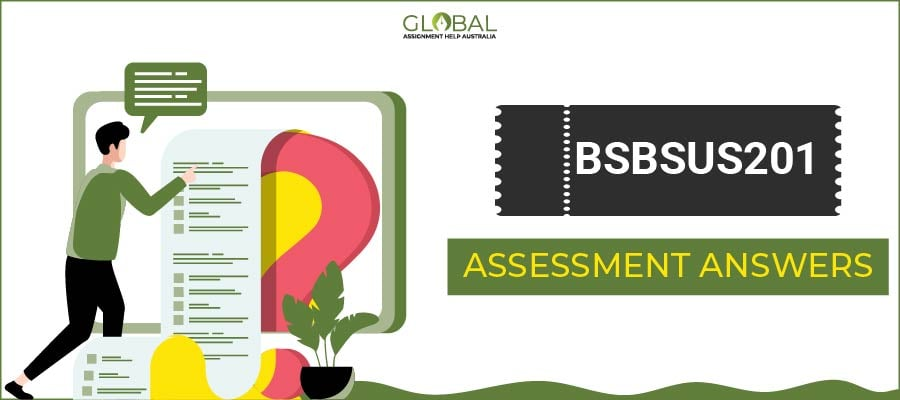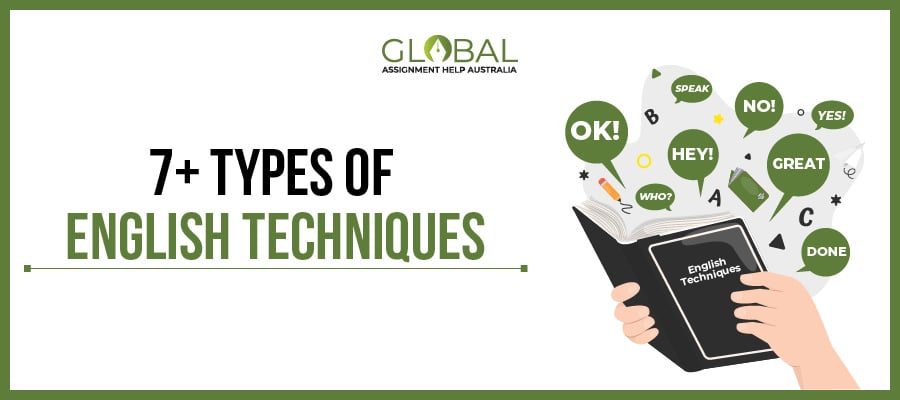0 Cart

# How to Master Trigonometry in Just 7 Simple Steps?

How to Master Trigonometry in Just 7 Simple Steps?

31 May 2019 3825 5 minutes

Share it :

Trigonometry is the branch of mathematics in which students study about triangles and cycles. It is a quite interesting fact that in order to learn a simple triangle it leads to so many applications including functions, and identities. The concept involved in trigonometry is not that much difficult but what makes this subject more challenging is that it is over-flooded with lots of identities and formulas. You learn all the four formulas and when you reach the fifth one, you can’t recall the previous ones. This is the actual problem that every student’s face.

So, are you also facing the same situation? If yes, then do not get panic.

Here are some of the tips suggested by the experts of Global Assignment Help Australia to learn these formulas and to beat the monster called trigonometry!

So, let's start to explore...

### How to Learn Trigonometry Functions?

We will start with the basic functions of trigonometry, i.e. sine, cos, and tan. The best way to learn this is to remember them in the following order.

#### Trigonometric Functions

 Sin Cos Tan OH AH OA Cosec Sec Cot
• You can also learn it like SOH, CAH, TOA which simply means:
Sin= opposite/hypotenuse
• Once you learn all these six formulas, then move ahead to know all the sign of trigonometric functions.

So, here is the best technique suggested by the experts of Global Assignment Help Australia to learn the trigonometric functions easily -

#### Signs of Trigonometric Functions

• 1st Quadrant = All functions are positive
• 2nd Quadrant = Only Sine and Cosec are positive, remaining all are negative.
• 3rd Quadrant = Similarly, Tangent and Cotangent are positive rest all are negative.
• 4th Quadrant = Sec and Cosine are positive rest all negative.

If you are still getting confused in remembering this, there is another tip discussed by our experts, that will help you remember the signs of trigonometric functions

Expert Tip: After School To College...here, the starting letter denotes the functions which are positive in their respective quadrant.

OK!!! so, once you learn all the signs of trigonometry, now let’s move ahead with the core of trigonometry, i.e. identities.

### 7 Tips to Learn Trigonometric Identities

When it comes to learn identities, students usually run away from it. They consider learning identities as a headache. So, to ease their burden, here are some tips from our experts that will definitely help you learn the identities of trigonometry in a easier manner:

Tip 1: Remember, instead of cramming the identities, try to understand it by relating the terms so that you can easily remember the formula.

Tip 2: ‘Sin’ always carries a negative sign, where ‘Cos’ carries a positive sign.

Tip3: You only need to learn any one sum identity, then you can easily derive the remaining sum identities. The technique you can use is ‘Sin x’ is an odd function and ‘Cos x’ is an even function.

Tip4: You cannot avoid Pythagorean identities and reciprocal identities because they are the main backbone of trigonometry.

Tip5: Always use the exponent rule to derive the identities and remember, sin x = (eix– e-ix)/2i and cos x = (eix+ e-ix)/2

Tip 6: In the subject like trigonometry, it is very important to have the basics clear because in case you forget the formula, only these basics can help you in recalling it.

Tip 7: Below given is the list of important identities that you should definitely learn so that you can easily complete the assignments and other tasks of trigonometry. They are:

• Pythagorean Identities
• Negative Angle Identities
• Co-functions Identities
• Trigonometric Functions Periodicity

So, by following these tips you can easily master the subject of trigonometry.

And finally, practice! practice! and practice!. Even after going through the above-mentioned tips, you feel like you are unable to handle the trigonometric problems and need expert’s help, then you may seek online trigonometry assignment help from us.

## Recent postLearn to Draft Best BSBSUS201 Assessment Answers Efficiently

If You Aim to Present Informational Solutions, Refer to This Blog to Accomplish This Essential Set of Information

08 Dec 2023 45 12 minutes Explore7+ Important English Techniques to Improve the Context

If You Want Formidable Fundamentals on English Techniques, Refer to This Blog to Achieve This Objective

05 Dec 2023 60 14 minutes ExploreGet Qualified TAE40116 Certificate IV in Training and Assessment

If You Want to Learn All About TAE40116 Assessment Answers, Then Referring to This Blog is a Beneficial Choice

04 Dec 2023 51 12 minutes Explore